Example Questions

Example Question #1 : Negative Numbers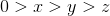Quantity A: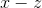Quantity B: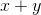Quantity A is greater

The relationship cannot be determined from the information given.

The two quantities are equal

Quantity B is greater

Quantity A is greater

Explanation:

Since both quantities have an, you can ignore this variable, which will give you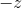andfor quantities A and B, respectively. Sinceandare both negative numbers,must be bigger than, which means that no matter what numbers they are, Quantity A must be bigger.

Example Question #2 : Negative Numbers

If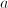andare integers such that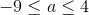and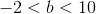, what is the smallest possible value of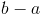?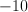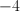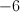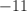Explanation:

To makeas small as possible, letbe as small as possible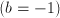, and subtract the largest value ofpossible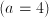: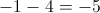Example Question #3 : Negative Numbers

Quantity A: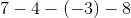Quantity B: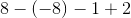Which of the following is true?

Quantity B is larger.

The two quantities are equal in size.

The relationship between the quantities cannot be determined.

Quantity A is larger.

Quantity B is larger.

Explanation:

A problem like this one is very easy.  All you need to do is manage your arithmetic well.  Remember that when you subtract a negative number, this is the same as adding the positive of that number.  Therefore, you can rewrite each of your quantities:

Quantity A: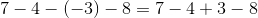Using a calculator, this comes out to be:Quantity B: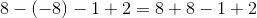Using a calculator, this comes out to be:Therefore, quantity B is larger.

Tired of practice problems?

Try live online GRE prep today.